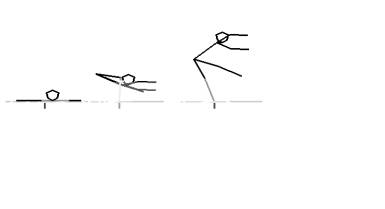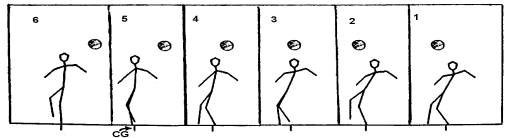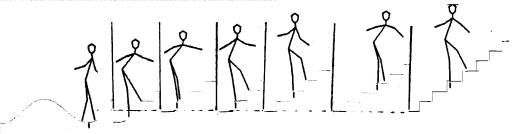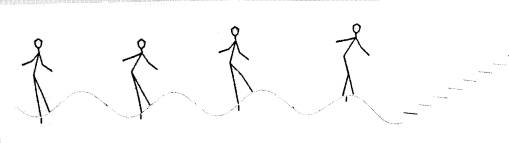## RENT A THINKER Home My Page Chat Tik-Tok 3        A Novel Algorithm for Simulation of A Walking ROBOT using Neural Network.

### 3.1       ABSTRACT

Multi layered neural networks are being applied in various fields of automation. One such application is developed for simulation of two legged Robot  to balance and walk using Neural Network based learning technique. Human limbs are simulated  and a skeleton of the human body is designed using computer graphics. A rule based  Neural Network Algorithm is developed to motivate the skeleton to walk. The motivation is created using a set of rules and an error functions to achieve the set goals. Some of the goals are like- keeping the Center of Gravity (CG) under the feet, keeping  the average height of the CG constant, spending minimum energy, traveling in a given direction etc.. Using this algorithm, the seven limb joints  of  the skeleton  are updated  and the limbs are plotted  iteratively to produce an animation effect of  walking. The ROBOT model is only inputted with the destination  co-ordinates, the actual movements of the limbs are generated automatically similar to human walking. The algorithm uses a technique similar to the gradient descendent method to minimize the error. Such algorithm may be applied to solve transportation problems in non-uniform space using multi legged ( wheel-less) vehicles.

### 3.2       INTRODUCTION

The nature has not provided wheels to the biological systems, however the most man made systems need wheels for transportation. Wheel preserves energy and is more efficient, provided it works on an uniform surface. Biological systems on the other hand can move on non-uniform  surfaces. The basic difference between biological and manmade system is that most of the manmade transportation  system  do not have sensory signal feed back with information processing capabilities.  In case of the transportation of the biological system, the eyes acquire the image of the environment, brain processes the image and computes the co-ordinate location, where the next step should be directed. While doing so, brain consider many aspects like -(a)  To keep the body in equilibrium by  keeping the CG under the feet, (b)  minimum change in the CG height to preserve the potential and kinetic energy, (c)  Considering  above two points, it calculates the maximum step size  based on the dimensions and the degree of freedom of the limbs, (d) the expected position of the step depending on the information received through eyes etc..

The brain continuously optimizes all the parameters based on different constraints as described above to move the body to the desired position. These processes are called walking, running, scrolling etc. and are very advance compared to any of the man made machines. To implement walking in wheel-less mobile systems- it is necessary to map the cinematic details to the processing and control unit so that an integrated control strategy is worked out in a multi limbed flexible systems for transportation. This type of control strategies are applicable to Robots, cranes, earthmovers, vehicles for distant planet etc.. This paper describes one such control strategy based on NN approach.

A model of human skeleton is simulated in two dimensional computer graphics. The simulation uses 10 limbs with proportionate length and weight. An algorithm is described to move the model on an artificial one-dimensional surface. The operation has several goals like keeping at least one leg on the surface at all the time, moving the body to the desired direction etc.. The goals are implemented using rule-based approach. The control signals are calculated for each limb depending on its current relative position and the desired goals. This is achieved iteratively by using an error function similar to FFNN [Rumalhert]. The sensitivity of the total error is calculated with respect to each limbs movement. The gradient descendent technique is used to update the incremental position of all the limbs. The following section describes the details of the walking algorithm and the simulation ‘C’ program. In the RESULTS section, some examples of behavior of the model are shown with the plots of model’s walking on different surfaces.

### 3.3       GRAPHICS SIMULATION

In this simulation, we have consider following ten limbs of human body - (a) neck (b) body (c) left arm1 (d) left arm2 (e) right arm1 (f) right arm2 (g) right thighs (h) right leg  (i) left thighs and (j) left leg. The fingers palms and feet are not included in the simulation; however, feet play an important role in balancing the body and walking.  It is interring to note that some people can walk on two sticks. Our simulation is similar to this. Each limb is considered as one dimensional line segment having length and weight proportional to that of the average human limbs.

A database is created for all the limbs each record has following details. Length, weight, absolute angle, maximum angle, minimum angle, driving end co-ordinates and other end co-ordinates. The maximum and minimum angles are having relative values and measured with respect to the limb where it is connected. A second database is used for the joints, which defines the connectivity of limbs with respect to each other.

The limbs are assembled at any time using above database. To place the model on a solid surface, the lowest tip of the limb is made to touch the surface by appropriately offsetting all the coordinates.  Initially all the absolute limb angles are initialized with random values.

#### 3.3.1     Standing on feet

The model is made to stand on its leg like human beings by maneuvering the position of the center of gravity. Center of Gravity (CG) of each limb is individually calculated and then the resultant co-ordinates of CG are calculated. To make the model stand, two constraints are imposed- (a) the average height of the CG with respect to the ground surface should be maintained and (b) the CG should be maintained between two feet. To achieve the above constraints, an iterative algorithm is developed, that minimizes the error of the CG. At the end of every iteration the limb angles are updated; limbs are reassembled and displayed on graphics screen to produce animation effect. To achieve the desire goal of CG constraints as described in section 2.1, there are may be possibilities of limb positions, e.g. the model could be upside down standing on its head and still achieve the desired CG position. There are other constraints added to the system. Some of them are (a) at least one of the leg should touch the ground surface at all the time, (b) no limb should go below ground surface (c) conserve the energy and (d) conserve the time.  The algorithm is based on minimizing the error of the primary goals using gradient descendent technique i.e use for training MLNN. The algorithm is described below -

#### 3.3.2     Algorithm for Standing

From a given position to the standing position, the model goes through a nonlinear translation of all the co-ordinates. During the translation, the model is restricted by the constraints like limiting angles, CG etc. The model works against gravitational field using the mussel forces, which drives the limb joints. To compute the locus of each limb, the error sensitivity of each limb is calculated. Depending on the magnetized and direction of these parameters, the limbs are moved proportionally. The movement is made proportional to an arbitrary constant ‘eta’, whose value is kept between 0.01 to 0.1. The sensitivity is calculated by calculating moving the limbs by a small angle in the direction and direction and have magnitudes as computed by the previous iteration.

#### 3.3.3     Walking Algorithm

In section 2.2, we have seen that how the model can stand on its feet from an arbitrary position. To make the robot walk, the model is given additional constraints to reach a given co-ordinates in standing posture. These constraints are  (a) minimizing the distance between the CG and the target location, (b) not dragging the body on the floor surface (c) keeping at least one feet on the ground surface.

### 3.4       RESULTS

The simulated model is tested in different environment. The model is brought to a random posture by choosing all the limb angles as random values and then letting a model to stand on its own and walk. The several frames are drawn between any two steps. each frame is drawn on alternate frame of the graphics. The pages are switched so that the drawing and display are pipelined to increase the display time.[Figure – 1(a)][Figure – 1(b)]

Figure-1 shows the capture picture of several frames from initial random position to walking.  The small vertical line below the surface represents the CG of each position.Figure -2 shows the model climbing to the staircase.In figure - 3, the model is tested on a irregular surface.

In all the experiments, the simulation shows case similarity of the model movement and human walking.

### 3.5       CONCLUSION

A computer graphics simulation of a human skeleton is made to perform walking by balancing the limbs and CG. The walking algorithm is based on gradient descendent technique. A neural network is trained with its inputs as the current angles of the limb and the direction of movements. The output of the network is trained with the next state of the corresponding angles. The network is trained using the data from the above model.

### 3.6       FUTURE WORK

It is possible to use the above algorithm for animation and automation in making of cartoon films.

### 3.7       REFERENCES

 William B. Gevarter, “ Intalligent Machines” ; an introductory perspective of Artificial  Intelligence and Robotics

 Ernest W. Kent, “ The Brains of Men and Machines “, BYTE-McGrow Hill.

 Michael A. Arbib, “ The Metaphorical Brain  “, an introduction to cybernetics as Artificial Intelligence and Brain theory

### 3.8    Published

Mazumdar Himanshu. S., and  Leena P.   "A novel algorithm for simulation of a (Two legged)  Robot  using  Neural  Network",   CSI Communications, ISSN 0970-647X, pp. 18-20, August, 1996.